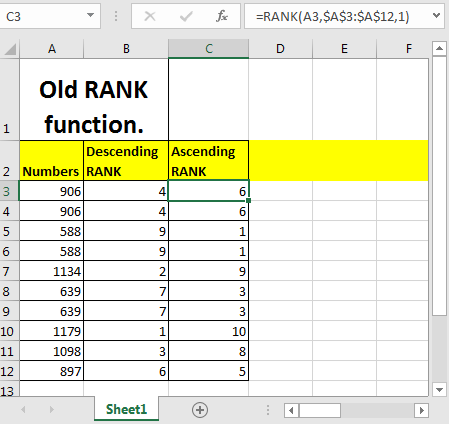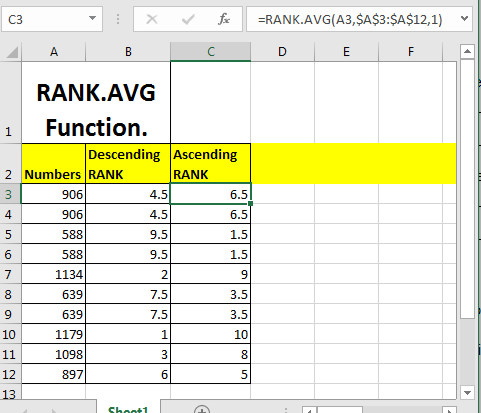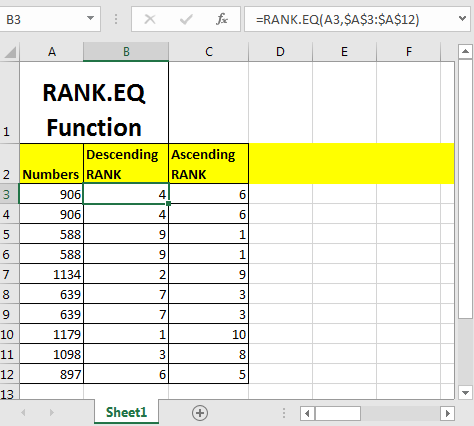# How to use the RANK function in ExcelIn this article, we will learn How to use the RANK function in Excel.

Rank of a value

In Excel, we are given a table and we need to set an index on the fixed column based on ascending or descending. Excel we call it ranking a value. The Rank Function in Excel is used to rank a number within a list. This function is outdated and Excel no longer recommends to use it. Excel has introduced two new ranking functions named RANK.AVG function and RANK.EQ function.  You can check them out by clicking the function. Let's understand the RANK function syntax and an example to illustrate the function usage.

RANK Function in Excel

The RANK function returns the rank of any value in the array index.

RANK Function syntax:

 =RANK(number,ref_range,[order])

Number: The number that you want to rank

Ref_Range: The range in which you want to rank. The number must be in the ref_range.

[Order]: This argument is optional. 1 is for  ascending ranking and 0 for descending. Ascending ranking means that higher number will get lover ranking. Descending ranking means higher number will get higher ranking. By default it is Descending.

Example :

All of these might be confusing to understand. Let's understand how to use the function using an example. So to rank each number in range A3:A12 in descending order we can write this RANK formula.

 =RANK(A3,\$A\$3:\$A\$12)

Similarly to rank each number in range A3:A12 in ascending order write this RANK formula.

 =RANK(A3,\$A\$3:\$A\$12,1)1 commands excel to rank in ascending order.

The best thing about the RANK function that you don’t need to sort the data to rank.

This RANK function was used extensively in Excel 2007 and earlier. Now in Excel 2016 RANK.AVG and RANK.EQ  are used.

RANK.AVG FUNCTION EXAMPLE

So to rank each number in range A3:A12 in descending order we can write this RANK.AVG formula.

 =RANK.AVG(A3,\$A\$3:\$A\$12)

Similarly to rank each number in range A3:A12 in ascending order write this RANK.AVG formula.

 =RANK.AVG(A3,\$A\$3:\$A\$12,1)1 commands excel to rank in ascending order.

The best thing about the RANK.AVG function is that you don’t need to sort the data to rank.

Since Excel 2010 till now RANK.AVG function is used to rank numbers with average ranking in excel.

RANK.EQ FUNCTION EXAMPLE

So to rank each number in range A3:A12 in descending order we can write this RANK.EQ formula.

 =RANK.EQ(A3,\$A\$3:\$A\$12)

Similarly to rank each number in range A3:A12 in ascending order write this RANK.EQ formula.

 =RANK.EQ(A3,\$A\$3:\$A\$12,1)1 commands excel to rank in ascending order.

The best thing about the RANK.EQ function is that you don’t need to sort the data to rank.

Since Excel 2010 till now RANK.EQ function is used to rank numbers with average ranking in excel.

Here are all the observational notes using the RANK function in Excel
Notes :

1. The function only works with numbers
2. The function only returns the rank of the chosen number. You can get the whole list by copying the formula from one cell and apply to all values in array.

Hope this article about How to use the RANK function in Excel is explanatory. Find more articles on calculating values and related Excel formulas here. If you liked our blogs, share it with your friends on Facebook. And also you can follow us on Twitter and Facebook. We would love to hear from you, do let us know how we can improve, complement or innovate our work and make it better for you. Write to us at info@exceltip.com.

Related Articles :

How to use the PERCENTILE function in Excel

How to use the PERCENTILE.INC function in Excel

How to use the PERCENTILE.EXC function in Excel

How to use the PERCENTRANK function in Excel

How to use the PERCENTRANK.INC function in Excel

How to use the PERCENTRANK.EXC function in Excel

How to use the QUARTILE function in Excel

How to use the QUARTILE.INC function in Excel

How to use the QUARTILE.EXC function in Excel

Popular Articles :

How to use the IF Function in Excel : The IF statement in Excel checks the condition and returns a specific value if the condition is TRUE or returns another specific value if FALSE.

How to use the VLOOKUP Function in Excel : This is one of the most used and popular functions of excel that is used to lookup value from different ranges and sheets.

How to use the SUMIF Function in Excel : This is another dashboard essential function. This helps you sum up values on specific conditions.

How to use the COUNTIF Function in Excel : Count values with conditions using this amazing function. You don't need to filter your data to count specific values. Countif function is essential to prepare your dashboard.

Terms and Conditions of use

The applications/code on this site are distributed as is and without warranties or liability. In no event shall the owner of the copyrights, or the authors of the applications/code be liable for any loss of profit, any problems or any damage resulting from the use or evaluation of the applications/code.